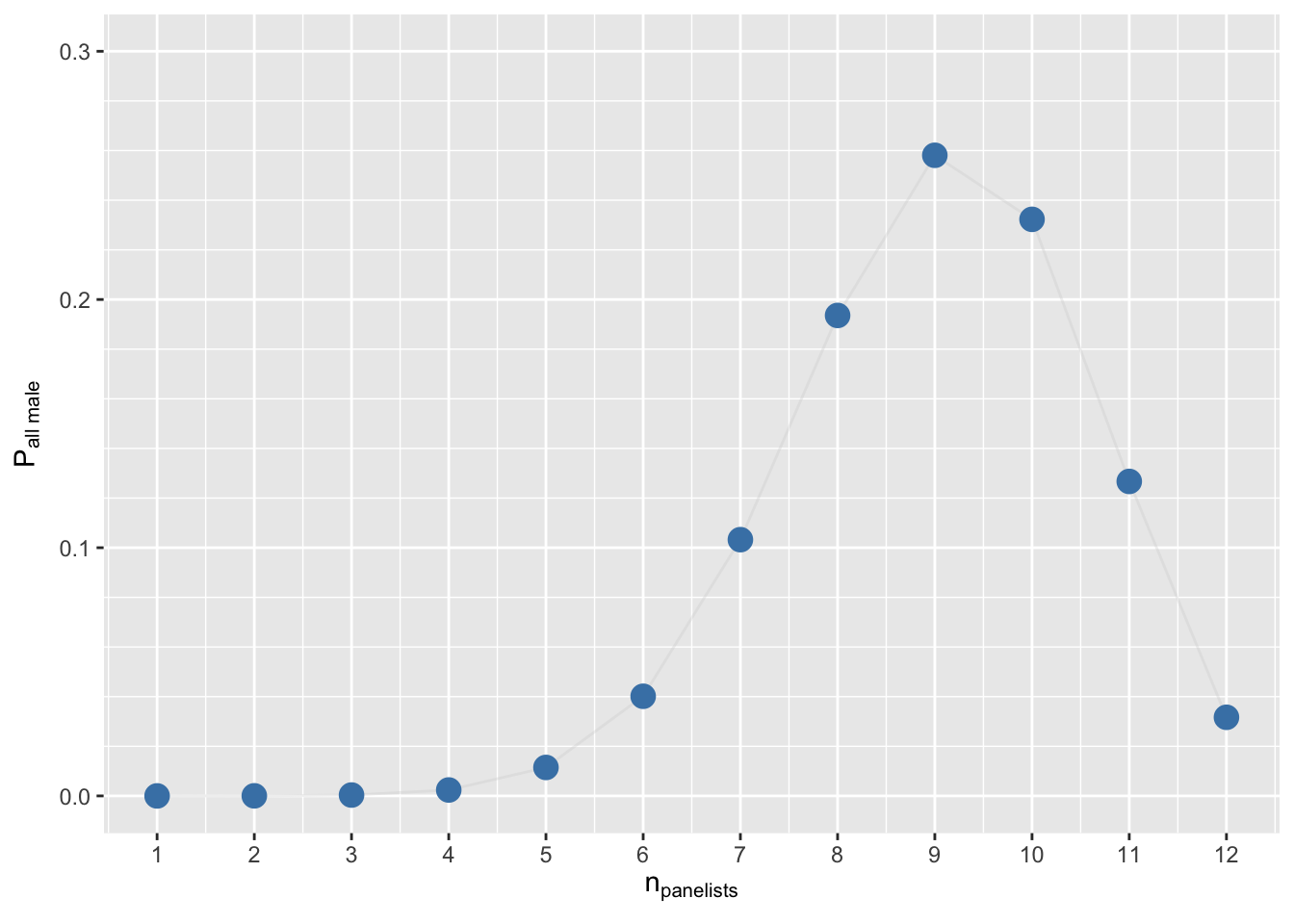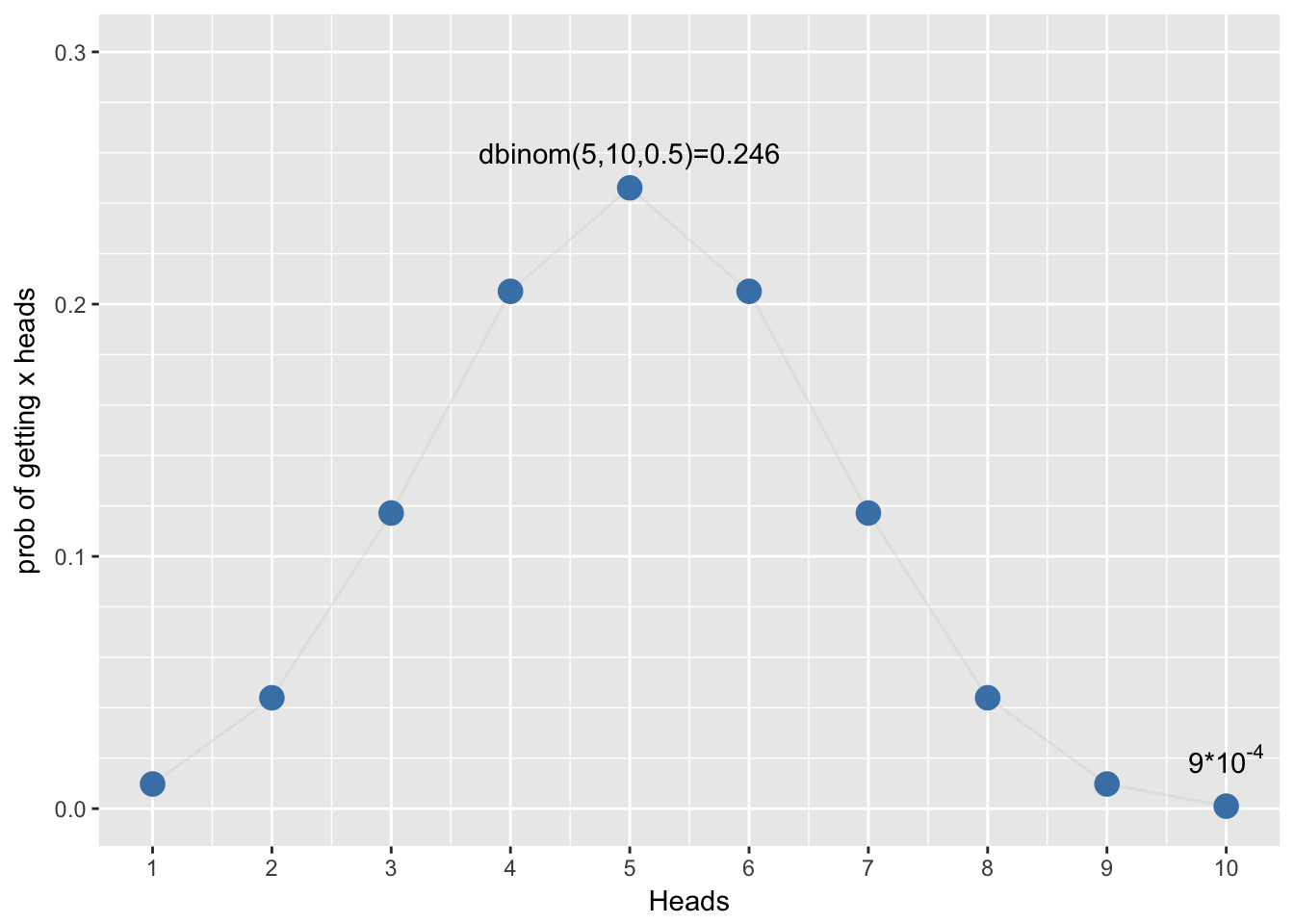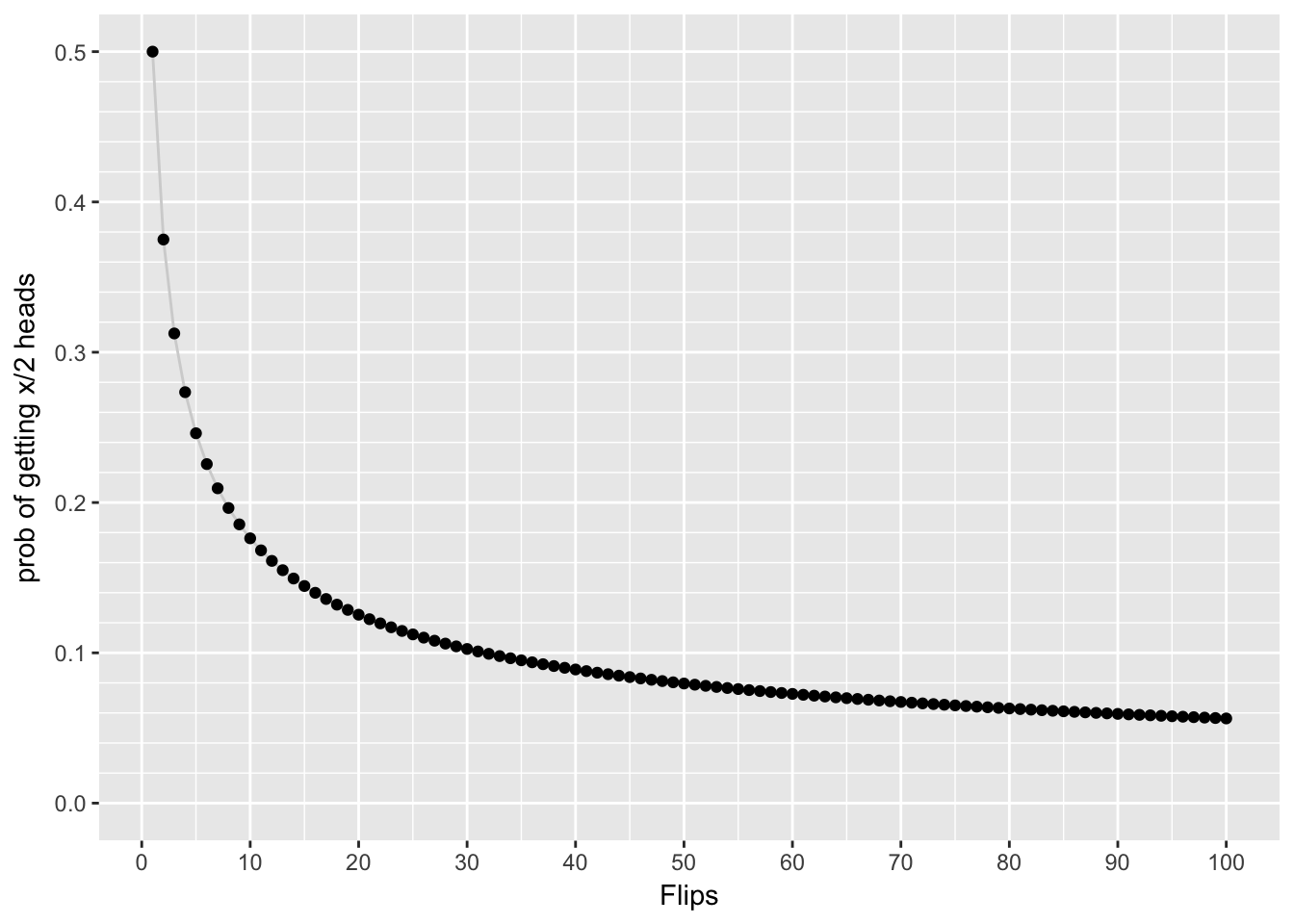# The binomial distribution

## Intro

If, say, 75% of “qualified” speakers are men, what’s the probability of 12 male panelists if random selection? (Source: sharon000)

library(latex2exp)
dbinom(12, size=12, prob = 0.75)
##  0.03167635
df <- data.frame(x=1:12, z=dbinom(1:12, size=12, prob = 0.75)); #df
ggplot(df)  +
geom_line(aes(x=x, y=z), color="lightgray", alpha=I(1/3)) +
geom_point(aes(x=x, y=z), size=4, color="Steelblue") +
scale_x_continuous(breaks=seq(1, 12, 1), name=TeX("n_{panelists}")) +
scale_y_continuous(limits = c(0,0.3), minor_breaks=seq(0, 0.3, 0.02), name=TeX("P_{all male}"))A coin is tossed 10 times. What is the probability of getting exactly 6 heads?

dbinom(6, 10, 0.5)
##  0.2050781
df <- data.frame(Heads=1:10, prob=dbinom(1:10, size=10, prob = 0.5)); #df
ggplot(df) + geom_line(aes(x=Heads, y=prob), color="lightgray", alpha=I(1/3)) +
scale_x_continuous(breaks=seq(1, 12, 1)) +
scale_y_continuous(limits = c(0,0.3), minor_breaks=seq(0, 0.3, 0.02), name="prob of getting x heads") +
annotate("text", x=5, y=0.26, label="dbinom(5,10,0.5)=0.246") +
#annotate("text", x=6, y=0.01, label="text[subscript]", parse=TRUE) +
annotate("text", x=10, y=0.02, label=TeX("$9*10^{-4}$", output='character'), parse=TRUE)1-pbinom(9,10,0.5)
##  0.0009765625
dbinom(2, 4, 0.5)  #less than the naïve estimate of 0.5
##  0.375
df <- data.frame(head=1:100, toss=seq(2, 200, 2)); #head(df)
df <- within (df, prob_head <- dbinom(head, toss, 0.5)); head(df)
##   head toss prob_head
## 1    1    2 0.5000000
## 2    2    4 0.3750000
## 3    3    6 0.3125000
## 4    4    8 0.2734375
## 5    5   10 0.2460938
## 6    6   12 0.2255859
ggplot(df) + geom_line(aes(x=head, y=prob_head), color="lightgray") + geom_point(aes(x=head, y=prob_head)) +
scale_x_continuous(breaks=seq(0, 100, 10), name="Flips") +
scale_y_continuous(limits = c(0,0.5), minor_breaks=seq(0, 0.5, 0.02), name="prob of getting x/2 heads")These are examples of the binomial distribution, one of the discrete distributions (the other important one being the Poisson distribution). It is used when there is a dichotomus outcome (success vs. failure).

## pmf

Its probability mass function is $f(x; n,P) = {n\choose x}P^x(1-P)^{n-x}$ where $${n\choose x}$$ is the binomial coefficient, a general way of selecting $$x$$ combinations from a total of $$n$$ things (‘$$n$$ choose $$x$$’) ${n\choose x} = \frac{n!}{x!(n-x)!}$

## Mean and variance

The expected value, or mean, of a Bernoulli trial is $$P$$ (the probability of success) and its variance is $$P(1-P)$$. The mean of a binomial distribution is $$nP$$ and its variance is $$nP(1-P)$$. Baguley

## Examples

80% of people who purchase pet insurance are women. If 9 pet insurance owners are randomly selected, find the probability that exactly 6 are women.

dbinom(6, 9, 0.8)
##  0.1761608

Rolling a die 8 times and getting 6 twice (versus a non-6, hence the binomial outcome)

dbinom(2,8,1/6)
##  0.2604762

You sell sandwiches. 70% of people choose chicken, the rest choose beef. What is the probability of selling 2 chicken sandwiches to the next 3 customers?

dbinom(2,3,0.7)
##  0.441

“70% choose chicken, so 7 of the next 10 customers should choose chicken” … what are the chances you are right?

dbinom(7,10,0.7)
##  0.2668279

0.27 so moral of the story: even though the long-run average is 70%, don’t expect 7 out of the next 10.

80% of adults with allergies report symptomatic relief with a specific medication. If the medication is given to 10 new patients with allergies, what is the probability that it is effective in exactly seven?

dbinom (7,10,0.8)
##  0.2013266

The likelihood that a patient with a heart attack dies of the attack is 0.04 (i.e., 4 of 100 die of the attack). Suppose we have 5 patients who suffer a heart attack, what is the probability that all will survive?

dbinom(0,5,0.04)
##  0.8153727

Suppose you flipped a coin 10 times (i.e., 10 trials), and the probability of getting “heads” was 0.5 (50%). What would be the probability of getting exactly 4 heads?

dbinom (4,10,0.5)
##  0.2050781

Cumulative probability of > 4 events

pbinom(4, 10, 0.5, lower.tail=FALSE)  #NOTE THAT WE USE pbinom, not dbinom
##  0.6230469

Cumulative probability of < 4 events

pbinom (3, 10, 0.5, lower.tail=TRUE)
##  0.171875